2022: SklogWiki celebrates 15 years on-line

# Critical points

The critical point, discovered in 1822 by Charles Cagniard de la Tour  , is a point found at the end of the liquid-vapour coexistence curve (the red point shown on the pressure-temperature plot on the right). At this point the temperature is known as the critical temperature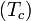$(T_c)$ and the pressure is known as the critical pressure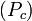$(P_c)$. For an interesting discourse on the "discovery" of the liquid-vapour critical point, the Bakerian Lecture of Thomas Andrews makes good reading . Critical points are singularities in the partition function. In the critical point vicinity (Ref.  Eq. 17a)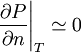$\left.\frac{\partial P}{\partial n}\right\vert_{T} \simeq 0$

and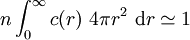$n \int_0^{\infty} c(r) ~4 \pi r^2 ~{\rm d}r \simeq 1$

For a review of the critical region see the work of Michael E. Fisher 

"... Turning now to the question of specific heats, it has long been known that real gases exhibit a large anomalous" specific-heat maximum above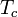$T_c$ which lies near the critical isochore and which is not expected on classical theory..."

also

"... measurements (Ref.  ) of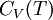$C_V(T)$ for argon along the critical isochore suggest strongly that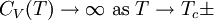$C_V(T) \rightarrow \infty ~{\rm as} ~ T \rightarrow T_c \pm$. Such a result is again inconsistent with classical theory."

Thus in the vicinity of the liquid-vapour critical point, both the isothermal compressibility and the heat capacity at constant pressure diverge to infinity.

## Solid-liquid critical point

It is widely held that there is no solid-liquid critical point. The reasoning behind this was given on the grounds of symmetry by Landau and Lifshitz . However, recent work using the Z2 potential suggests that this may not be the last word on the subject. .

## Critical exponents

Main article: Critical exponents

## Yang-Yang anomaly

Main article: Yang-Yang anomaly